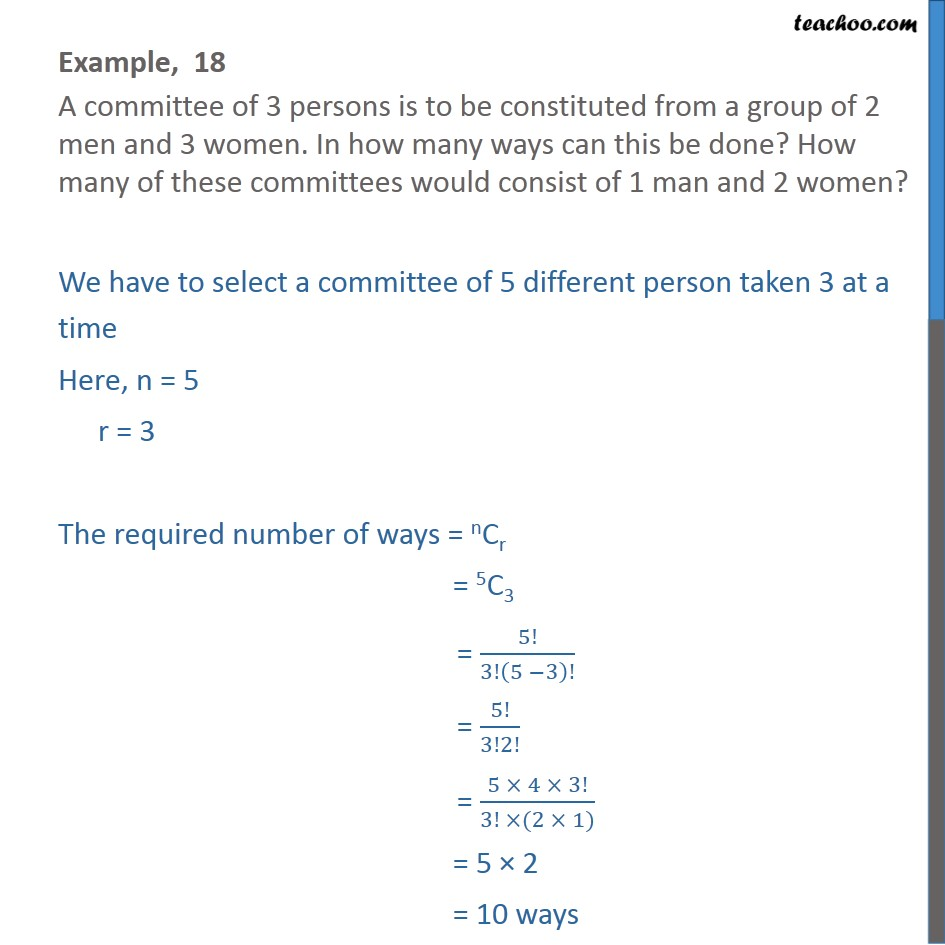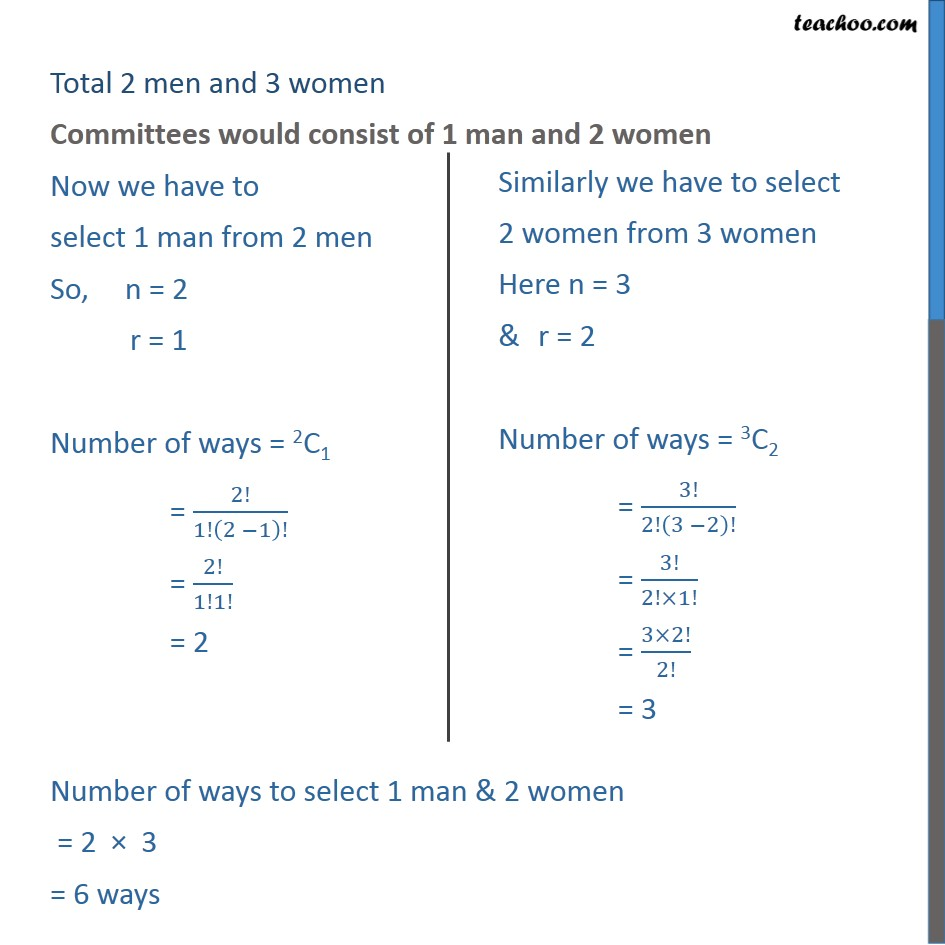1. Chapter 7 Class 11 Permutations and Combinations (Term 2)
2. Serial order wise
3. Examples

Transcript

Example, 18 A committee of 3 persons is to be constituted from a group of 2 men and 3 women. In how many ways can this be done? How many of these committees would consist of 1 man and 2 women? We have to select a committee of 5 different person taken 3 at a time Here, n = 5 r = 3 The required number of ways = nCr = 5C3 = 5!/3!(5 −3)! = 5!/3!2! = (5 × 4 × 3!)/(3! ×(2 × 1)) = 5 × 2 = 10 ways Total 2 men and 3 women Committees would consist of 1 man and 2 women Now we have to select 1 man from 2 men So, n = 2 r = 1 Number of ways = 2C1 = 2!/1!(2 −1)! = 2!/1!1! = 2 Number of ways to select 1 man & 2 women = 2 × 3 = 6 ways